Olympiad Test Level 1: Multiplication and Division - 1

# Olympiad Test Level 1: Multiplication and Division - 1

Test Description

## 10 Questions MCQ Test Math Olympiad for Class 2 | Olympiad Test Level 1: Multiplication and Division - 1

Olympiad Test Level 1: Multiplication and Division - 1 for Class 2 2022 is part of Math Olympiad for Class 2 preparation. The Olympiad Test Level 1: Multiplication and Division - 1 questions and answers have been prepared according to the Class 2 exam syllabus.The Olympiad Test Level 1: Multiplication and Division - 1 MCQs are made for Class 2 2022 Exam. Find important definitions, questions, notes, meanings, examples, exercises, MCQs and online tests for Olympiad Test Level 1: Multiplication and Division - 1 below.
Solutions of Olympiad Test Level 1: Multiplication and Division - 1 questions in English are available as part of our Math Olympiad for Class 2 for Class 2 & Olympiad Test Level 1: Multiplication and Division - 1 solutions in Hindi for Math Olympiad for Class 2 course. Download more important topics, notes, lectures and mock test series for Class 2 Exam by signing up for free. Attempt Olympiad Test Level 1: Multiplication and Division - 1 | 10 questions in 20 minutes | Mock test for Class 2 preparation | Free important questions MCQ to study Math Olympiad for Class 2 for Class 2 Exam | Download free PDF with solutions
 1 Crore+ students have signed up on EduRev. Have you?
Olympiad Test Level 1: Multiplication and Division - 1 - Question 1

### There are 5 apples hanging on one tree. How many apples are there on 7 such trees?

Detailed Solution for Olympiad Test Level 1: Multiplication and Division - 1 - Question 1

Number of apples hanging one tree are = 5
Number of apples hanging on 7 trees = 7 × 5 = 35

Olympiad Test Level 1: Multiplication and Division - 1 - Question 2

### Total number of erasers in the figure shown below is equal to the product of _________.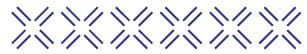Detailed Solution for Olympiad Test Level 1: Multiplication and Division - 1 - Question 2

Number of erasers in one cross (×) = 4
Number of crosses = 6
Total number of erasers in the figure = 4 × 6 = 24

Olympiad Test Level 1: Multiplication and Division - 1 - Question 3

### Which of the number in the figure shows the maximum value?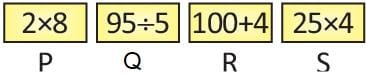Detailed Solution for Olympiad Test Level 1: Multiplication and Division - 1 - Question 3

R is maximum R = 100 + 4 = 104

Olympiad Test Level 1: Multiplication and Division - 1 - Question 4

If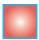= 6, which one of the following is correct for 30?

Detailed Solution for Olympiad Test Level 1: Multiplication and Division - 1 - Question 4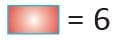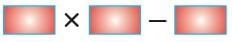= 6 × 6 – 6 = 30

Olympiad Test Level 1: Multiplication and Division - 1 - Question 5

Ifstands for 24, thenstands for:

Detailed Solution for Olympiad Test Level 1: Multiplication and Division - 1 - Question 5= 24
3= 24= 24 ÷ 3
= 8

Olympiad Test Level 1: Multiplication and Division - 1 - Question 6

Divide the product of 14 and 12 by 8.

Detailed Solution for Olympiad Test Level 1: Multiplication and Division - 1 - Question 6

Product of 14 and 12 = 14 × 12 = 168
Now, divide it by 8 = 168 ÷ 8 = 21

Olympiad Test Level 1: Multiplication and Division - 1 - Question 7

25 × 2 × 3 is equal to:

Detailed Solution for Olympiad Test Level 1: Multiplication and Division - 1 - Question 7

25 × 2 × 3 = 50 × 3 = 150

Olympiad Test Level 1: Multiplication and Division - 1 - Question 8

There are 112 ice-creams. These ice creams should be equally divided among 7 girls, how many ice creams each girl will get?

Detailed Solution for Olympiad Test Level 1: Multiplication and Division - 1 - Question 8

Total number of ice-creams = 112
No. of girls = 7
No. of ice-creams will each girl get
= 112 ÷ 7 = 16

Olympiad Test Level 1: Multiplication and Division - 1 - Question 9

In a class, 2 students can sit on a bench. How many students can sit on 13 such benches?

Detailed Solution for Olympiad Test Level 1: Multiplication and Division - 1 - Question 9

Total number of students who can sit on 13 benches = 2 × 13 = 26

Olympiad Test Level 1: Multiplication and Division - 1 - Question 10

3 + 12 = .................... × 3

Detailed Solution for Olympiad Test Level 1: Multiplication and Division - 1 - Question 10

3 + 12 = 15
..... × 3 = 15
..... = 15 ÷ 3
..... = 5

## Math Olympiad for Class 2

72 tests
 Use Code STAYHOME200 and get INR 200 additional OFF Use Coupon Code
Information about Olympiad Test Level 1: Multiplication and Division - 1 Page
In this test you can find the Exam questions for Olympiad Test Level 1: Multiplication and Division - 1 solved & explained in the simplest way possible. Besides giving Questions and answers for Olympiad Test Level 1: Multiplication and Division - 1, EduRev gives you an ample number of Online tests for practice

72 tests